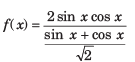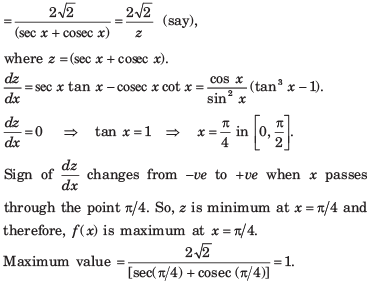Courses

# Test: Differential Calculus

## 20 Questions MCQ Test RRB JE for Electronics & Communication Engineering | Test: Differential Calculus

Description
This mock test of Test: Differential Calculus for GATE helps you for every GATE entrance exam. This contains 20 Multiple Choice Questions for GATE Test: Differential Calculus (mcq) to study with solutions a complete question bank. The solved questions answers in this Test: Differential Calculus quiz give you a good mix of easy questions and tough questions. GATE students definitely take this Test: Differential Calculus exercise for a better result in the exam. You can find other Test: Differential Calculus extra questions, long questions & short questions for GATE on EduRev as well by searching above.
QUESTION: 1

### If u = xm yn  then

Solution:

Given that u = xyn

Taking logarithm of both sides, we get log u = m log x + n log y Differentiating with respect to x,we get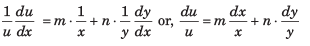QUESTION: 2

###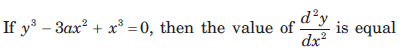​ ​ ​  to

Solution: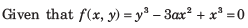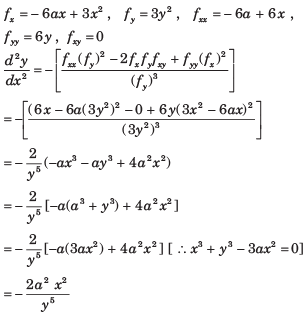QUESTION: 3

###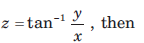​ ​ ​

Solution: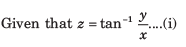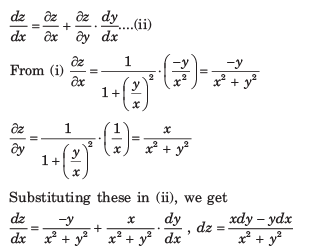QUESTION: 4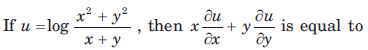Solution: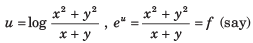f is a homogeneous function of degree one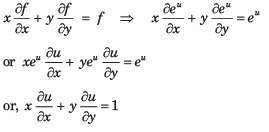QUESTION: 5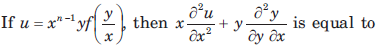Solution: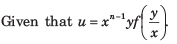It is a homogeneous function of degree n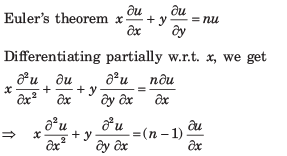QUESTION: 6

Match the List–I with List–II.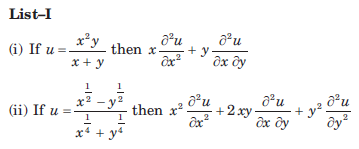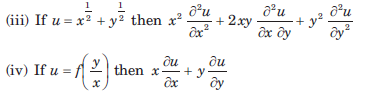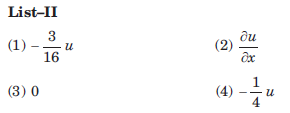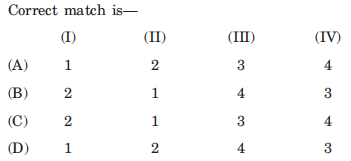Solution: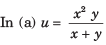It is a homogeneous function of
degree 2.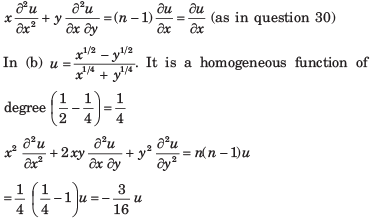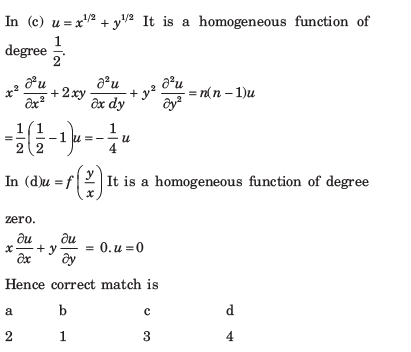QUESTION: 7

If an error of 1% is made in measuring the major and minor axes of an ellipse, then the percentage error in the area is approximately equal to

Solution:

Let 2 a and 2 b be the major and minor axes of the ellipse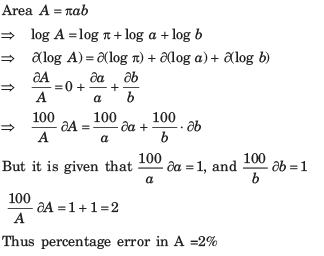QUESTION: 8

Consider the Assertion (A) and Reason (R) given below: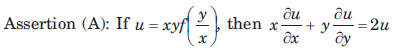Reason (R): Given function u is homogeneous of degree 2 in x and y.
Of these statements

Solution:

Given that  u = xyf(y/x) Since it is a homogeneous function of degree 2.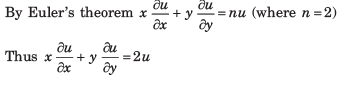QUESTION: 9

If u = x log xy, where x3 + y3 + 3xy = 1, then du/dx is equal to

Solution:

Given that u = x log xy ... (i)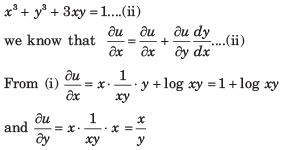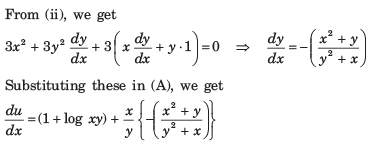QUESTION: 10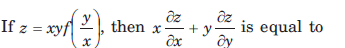Solution:

The given function is homogeneous of degree 2.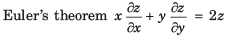QUESTION: 11

If a < 0, then f(x) = eax + e-ax is decreasing for

Solution: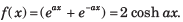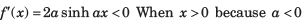QUESTION: 12

f(x) = x2e-x is increasing in the interval

Solution: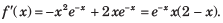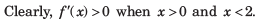QUESTION: 13

The least value of a for which f(x) = x2 + ax + 1 is increasing on ] 1, 2, [ is

Solution:

f'(x) = (2x + a)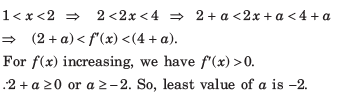QUESTION: 14

The minimum distance from the point (4, 2) to the parabola y2 =​ 8x is

Solution:

Let the point closest to (4, 2) be (2t2,4)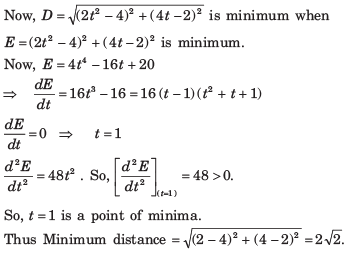QUESTION: 15

The co-ordinates of the point on the parabola y = x2 + 7x + 2 which is closest to the straight line y = 3x - 3, are

Solution:

Let the required point be P(x, y). Then, perpendicular distance of P(x, y) from y -  3x - 3 = 0 is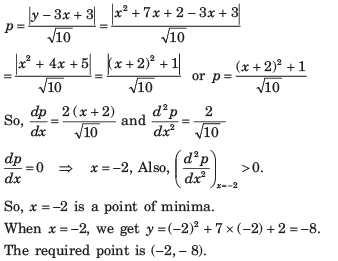QUESTION: 16

The shortest distance of the point (0, c), where 0 ≤ c ≤ 5, from the parabola y = x2 is

Solution:

Let A (0,c) be the given point and P (x, y) be any point on y = x2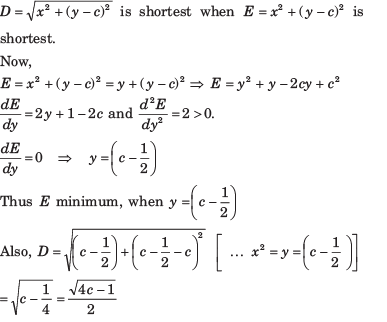QUESTION: 17

The maximum value of ( 1/x)x is

Solution: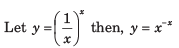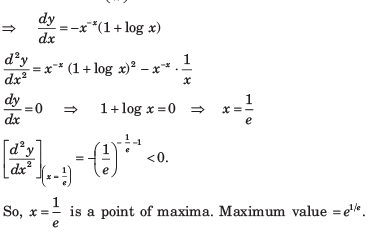QUESTION: 18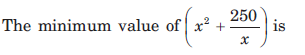Solution: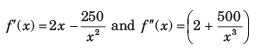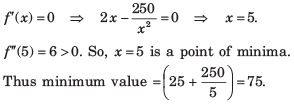QUESTION: 19

The maximum value of f ( x) = (1 + cos x) sin x is

Solution: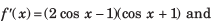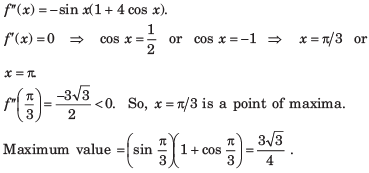QUESTION: 20

The greatest value of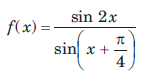on the interval [0, π/2] is

Solution: